# Loglogistic Distribution Example

This example appears in the Life Data Analysis Reference book.

Determine the loglogistic parameter estimates for the data given in the following table.$\overset{{}}{\mathop{\text{Test data}}}\,\,\!$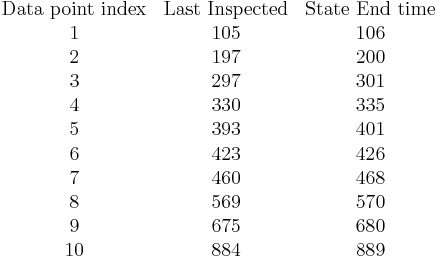$\begin{matrix} \text{Data point index} & \text{Last Inspected} & \text{State End time} \\ \text{1} & \text{105} & \text{106} \\ \text{2} & \text{197} & \text{200} \\ \text{3} & \text{297} & \text{301} \\ \text{4} & \text{330} & \text{335} \\ \text{5} & \text{393} & \text{401} \\ \text{6} & \text{423} & \text{426} \\ \text{7} & \text{460} & \text{468} \\ \text{8} & \text{569} & \text{570} \\ \text{9} & \text{675} & \text{680} \\ \text{10} & \text{884} & \text{889} \\ \end{matrix}\,\!$

Set up the folio for times-to-failure data that includes interval and left censored data, then enter the data. The computed parameters for maximum likelihood are calculated to be: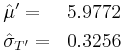\begin{align} & {{{\hat{\mu }}}^{\prime }}= & 5.9772 \\ & {{{\hat{\sigma }}}_{{{T}'}}}= & 0.3256 \end{align}\,\!

For rank regression on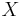$X\,\!$: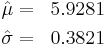\begin{align} & \hat{\mu }= & 5.9281 \\ & \hat{\sigma }= & 0.3821 \end{align}\,\!

For rank regression on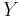$Y\,\!$: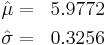\begin{align} & \hat{\mu }= & 5.9772 \\ & \hat{\sigma }= & 0.3256 \end{align}\,\!